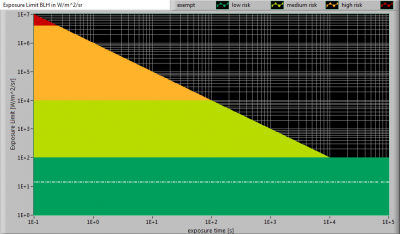# OliNo

Renewable Energy

## SALED – LED Tube 60cm 6000KSALED presents an led tube. The measurements from OliNo show that the lamp emits a cold white light with a color temperature of 6181 K. The lamp consumes 8.9 W and has a luminous flux of 907 lm. This results in an efficacy of 102 lm/W. The lamps energy category label is A+.

This article shows the measurement results. Many parameters are also found in the Eulumdat file.

See this overview for a comparison with other light bulbs.

### Summary measurement data

parameter meas. result remark
Color temperature 6181 K cold white
Luminous intensity Iv 248.8 Cd Measured straight underneath the lamp.
Illuminance modulation index 19 % Measured with a light sensor looking at the lamp (angle not defined). Is a measure for the amount of flickering.
Beam angle 135 deg 135 deg is the beam angle for the C0-C180-plane (perpendicular to the length direction of the lamp) and 111 deg is the beam angle for the C90-C270 plane, which is along the length direction of the lamp.
Power Factor 0.91 An electrical load with this power factor means that for every 1 kWh net energy consumed, there has been 0.45 kVAhr for reactive energy.
THD 26 % Total Harmonic Distortion.
Max inrush current 0.135 A This current has been found at a voltage start angle of 100 degrees.
Luminous flux 907 lm
Luminous efficacy 102 lm/W
EU2013-label classification A+ The energy class, from A++ (more efficient) to E (least efficient). This label is an update of the previous version, and compulsory from Sept 2013.
CRI_Ra 73 Color Rendering Index.
Coordinates chromaticity diagram x=0.3188 en y=0.3294
Fitting FL-tube This tube is powered from one side.
PAR-value 2.3 uMol/s/m2 The number of photons seen by an average plant when it is lit by the light of this light bulb. Value valid at 1 m distance from light bulb.
PAR-photon efficacy 0.9 uMol/s/We The total emitted number of photons by this light, divided by its consumption in W. It indicates a kind of efficacy in generating photons.
Photon current 13.4 uMol/s The total number of photons in the light of this lamp.
S/P ratio 2.0 This factor indicates the amount of times more efficient the light of this light bulb is perceived under scotopic circumstances (low environmental light level).
L x W x H external dimensions 588 mm x 33 mm x 33 mm External dimensions of the lamp.
L x W x H luminous area 540 mm x 30 mm x 15 mm Dimensions of the luminous area (used in Eulumdat file). It is the surface of the white opal cover.
General remarks The ambient temperature during the whole set of illuminance measurements was 27.1 – 28.0 deg C.

The temperature of the housing gets maximally about 14 degrees hotter than ambient temperature.

Warm up effect: During the warmup time the illuminance doesn’t vary significantly (< 5 %).

During the warmup time the power doesn’t vary significantly (< 5 %).

Voltage dependency: There is no (significant) dependency of the illuminance when the power voltage varies between 200 – 250 V AC.
There is no (significant) dependency of the consumed power when the power voltage varies between 200 – 250 V AC.

At the end of the article an additional photo.

Dimmable no Info from manufacturer.

Biologic effect factor
0.767 According to pre-norm DIN V 5031-100:2009-06.
Blue Light Hazard risk group 0 0=exempt, 1=low, 2 = moderate, 3=high risk.
Eulumdat fileRight click on icon and save the file.

### Overview table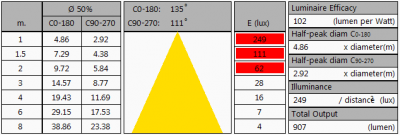The overview table is explained on the OliNo website.

Please note that this overview table makes use of calculations, use this data with care as explained on the OliNo site. E (lux) values are not accurate, when within 5 x 541 mm (maximal luminous size, eventually diagonally measured)= 2705 mm. Within this distance from the lamp (data given in red), the measured lux values will be less than the computed values in this overview as the measurements are then within the near field of the lamp.

### EU 2013 Energy label classification

Important for the energy classification are the corrected rated power and the useful luminous flux.
The measured rated power is 8.9 W and might need to be corrected. The correction is dependent from the lamp type and whether or not the lamp control gear is included or not. The choice for this lamp is the following classification: Lamps with own control gear (external or internal). As a result the corrected rated power becomes: 8.9 W.
The luminous flux measured is 907 lm. The classification of this lamp needed to determine the useful flux is: Non-directional lamps. Then the useful flux becomes 907 lm. Now a reference power can be calculated.

The energy efficiency coefficient is P_corr / P_ref = 0.13.Zip file with 6 EU energy labels of this lamp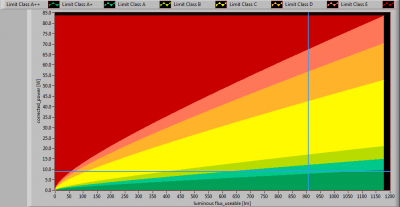The lamp’s performance in the lumen-Watt field, with the energy efficacy fields indicated.

### Eulumdat light diagram

This light diagram below comes from the program Qlumedit, that extracts these diagrams from an Eulumdat file. This light diagram is explained on the OliNo site.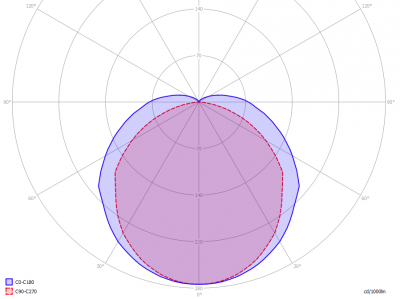The light diagram giving the radiation pattern.

The light diagram indicates the beam in the C0-C180 plane (perpendicular to the length direction of the lamp) and in the plane perpendicular to that, the C90-C270 plane (along the length direction of the lamp).

### Illuminance Ev at 1 m distance, or luminous intensity Iv

Herewith the plot of the averaged luminous intensity Iv as a function of the inclination angle with the light bulb.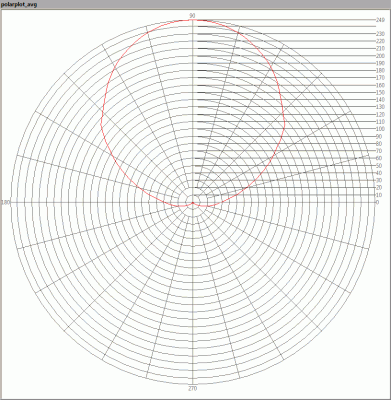The radiation pattern of the light bulb.

This radiation pattern is the average of the light output of the light diagram given earlier. Also, in this graph the luminous intensity is given in Cd.

These averaged values are used (later) to compute the lumen output.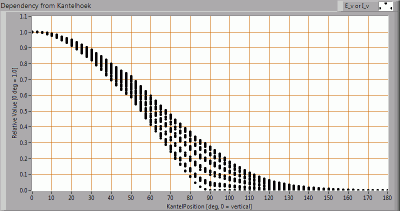Intensity data of every measured turn angle at each inclination angle.

This plot shows per inclination angle the intensity measurement results for each turn angle at that inclination angle. There normally are differences in illuminance values for different turn angles. However for further calculations the averaged values will be used.

When using the average values per inclination angle, the beam angle can be computed, being 135 deg for the C0-C180 plane and 111 deg for the C90-C270 plane.

### Luminous flux

With the averaged illuminance data at 1 m distance, taken from the graph showing the averaged radiation pattern, it is possible to compute the luminous flux.

The result of this computation for this light spot is a luminous flux of 907 lm.

### Luminous efficacy

The luminous flux being 907 lm, and the consumed power of the lamp being 8.9 Watt, results in a luminous efficacy of 102 lm/Watt.

### Electrical properties

The power factor is 0.91. An electrical load with this power factor means that for every 1 kWh net energy consumed, there has been 0.45 kVAhr for reactive energy.

 Lamp voltage 229.90 V Lamp current 0.043 A Power P 8.9 W Apparent power S 9.8 VA Power factor 0.91

Of this lamp the voltage across and the resulting current through it are measured and graphed. See the UI acquisition on the OliNo site how this is obtained.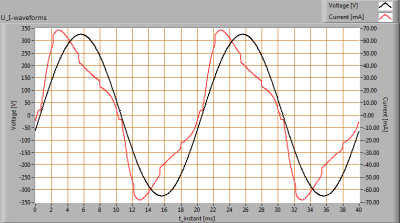Voltage across and current through the lightbulb

This current waveform has been checked on requirements posed by the norm IEC 61000-3-2:2006 (including up to A2:2009). See also the IEC 61000-3-2:2006 explanation on the OliNo website.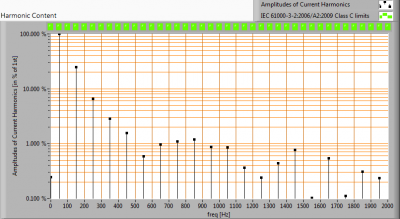Harmonics in the current waveform and checked against IEC61000-3-2:2006 and A2:2009

When the consumed power is <= 25 W there are no limits for the harmonics.

The Total Harmonic Distortion of the current is computed and its value is 26 %.

### Inrush current

The inrush current has been measured for different voltage start angles; from 0 – 170 degrees with a 10 degrees step. The current- and voltage values have been acquires at a sample speed of 39.9 kS/s. Then this data has been fed into a second order 2kHz low pass Butterworth filter. This removes the current spikes that do not represent relevant values.
The lamp was two minutes off before every inrush current measurement was made.

 Test voltage 230.0 V Frequency of the voltage 50.0 Hz Maximum inrush current 0.135 A This current has been found at a voltage start angle of 100 degrees. Minimal inrush current 0.016 A This current has been found at a voltage start angle of 0 degrees.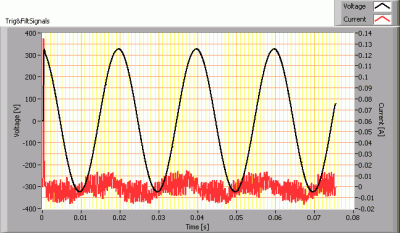Inrush current found at worst-case voltage start angleFirst cycle of the maximum inrush current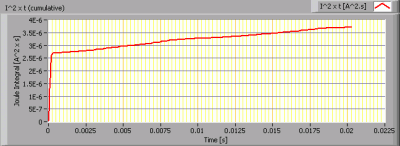The energy I2t during the first 10 ms of the first current cycle

### Temperature measurements lampTemperature image(s).

 status lamp > 2 hours on ambient temperature 26 deg C reflected background temperature 26 deg C camera Flir T335 emissivity 0.95 measurement distance 1 m IFOVgeometric 0.136 mm per 0.1 m distance NETD (thermal sensitivity) 50 mK

### Color temperature and Spectral power distribution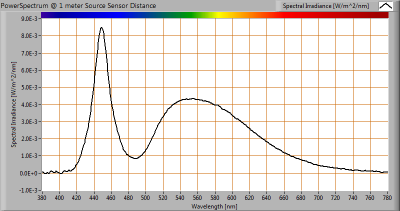The spectral power distribution of this light bulb, energies on y-axis valid at 1 m distance.

The measured color temperature is 6181 K which is cold white.

This color temperature is measured straight underneath the light bulb. Below a graph showing the color temperature for different inclination angles.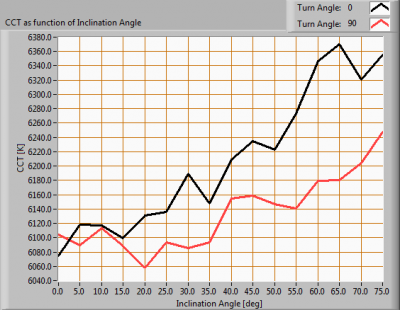Color temperature as a function of inclination angle.

The color temperature is given for inclination angles up to 75 deg. Beyond that value the illuminance is so low (< 5 lux) that it has not been used for color determination of the light.

For the C0-C180 plane: the beam angle of 135 deg is equivalent to 67.6 deg inclination angle,which is the area where most of the light falls within. The maximum variation of color temperature in this inclination area is about 1 %.

For the C90-C270 plane: the beam angle of 111 deg is equivalent to 55.6 deg inclination angle,which is the area where most of the light falls within. The maximum variation of color temperature in this inclination area is about 3 %.

### PAR value and PAR spectrum

To make a statement how well the light of this light bulb is for growing plants, the PAR-area needs to be determined. See the explanation about PAR on the OliNo website how this all is determined and the explanation of the graph.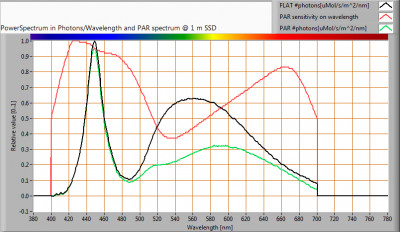The photon spectrum, then the sensitivity curve and as result the final PAR spectrum of the light of this light bulb

parameter value unit
PAR number 2.3 uMol/s/m^2
PAR photon current 8.4 uMol/s
PAR photon efficacy 0.9 uMol/s/W

The PAR efficiency is 65 % (valid for the PAR wave length range of 400 – 700 nm). This is the maximum percentage of the total of photons in the light that is effectively used by the average plant (since the plant might not take 100 % of the photons at the frequency where its relative sensitivity is 100 %).

### S/P ratio

The S/P ratio and measurement is explained on the OliNo website. Here the results are given.The power spectrum, sensitivity curves and resulting scotopic and photopic spectra (spectra energy content defined at 1 m distance).

The S/P ratio of the light coming from this lamp is 2.0.

More info on S/P ratio can be found on the OliNo website.

### Chromaticity diagram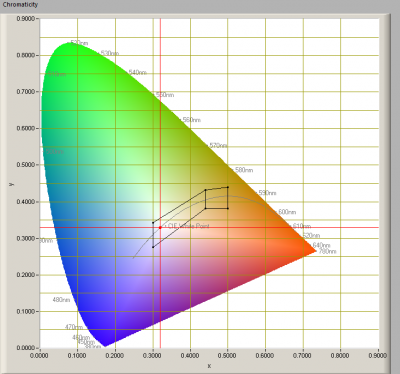The chromaticity space and the position of the lamp’s color coordinates in it.

The point of the light in this diagram is inside the area indicated with class A. This area indicates an area for signal lamps, see also the article on signal lamps and color areas on the OliNo website.

The color coordinates are x=0.3188 and y=0.3294.

### Color Rendering Index (CRI) or also Ra

Herewith the image showing the CRI as well as how well different colors are represented (rendered). The higher the number, the better the resemblance with the color when a black body radiator would have been used (the sun, or an incandescent lamp). Practical information and also some critics about the CRI can be found on the OliNo website.

Each color has an index Rx, and the first 8 indexes (R1 .. R8) are averaged to compute the Ra which is equivalent to the CRI.CRI of the light of this lightbulb.

This value of 73 indicates how well the light of this lamp can render well a set of reference colors, this in comparison with the light of a reference source (for color temperatures < 5000K a black radiator is used as reference and for color temperatures > 5000K the sun or the light outside during the day).

The value of 73 is smaller than the value of 80 that is considered as a minimum for working areas in general.

Note: the chromaticity difference is 0.0027 and indicates the distance to the Planckian Locus. There is a value mentioned of max 5.4E-3 in section 5.3 of CIE 13.3-1995 however not further explanation of it.
An other reference with signal lights as a reference is given in the chromaticity diagram.

### Voltage dependency

The dependency of a number of lamp parameters on the lamp voltage is determined. For this, the lamp voltage has been varied and its effect on the following light bulb parameters measured: illuminance E_v [lx], the lamppower P [W] and the luminous efficacy [lm/W] (this latter is estimated here by dividing the found E_v value by P).Lamp voltage dependencies of certain light bulb parameters

There is no (significant) dependency of the illuminance when the power voltage varies between 200 – 250 V AC.
There is no (significant) dependency of the consumed power when the power voltage varies between 200 – 250 V AC.

When the voltage varies abruptly with + or – 5 V AC then this results in a variation of the illuminance of maximally 0.2 %. This difference in illuminance is not visible (when it occurs abruptly).

### Warm up effects

After switch on of a cold lamp, the effect of heating up of the lamp is measured on illuminance E_v [lx], the lamppower P [W] and the luminous efficacy [lm/W].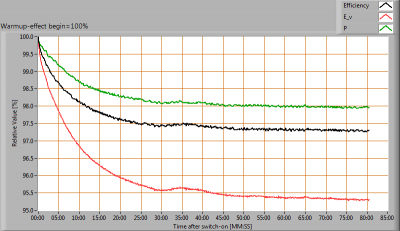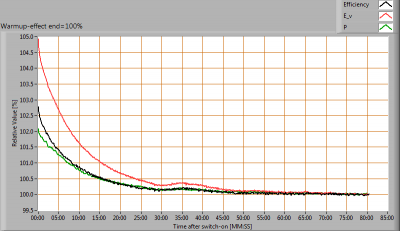Effect of warming up on different light bulb parameters. In the first graph the 100 % level is put at begin, and in the last graph the 100 % level is put at the end.

During the warmup time the illuminance doesn’t vary significantly (< 5 %).

During the warmup time the power doesn’t vary significantly (< 5 %).

### Measure of flickering

An analysis is done on the measure of flickering of the light output by this light bulb. See the article on flickering on OliNo site for more information.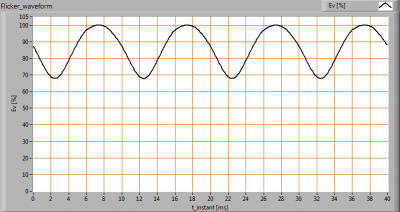The measure of fast illuminance variation of the light of the light bulb

parameter value unit
Flicker frequency 100.0 Hz
Illuminance modulation index 19 %

The illuminance modulation index is computed as: (max_Ev – min_Ev) / (max_Ev + min_Ev).

### Biologic effect

The biologic effect shows the level of impact the light of this lamp can have on the day-night rhythm of human beings (as well as the suppression of melatonin production). See also the explanatory article on biologic effect on OliNo.
The important parameters (according to prenorm DIN V 5031-100:2009-06):

 biologic effect factor 0.767 kbiol trans (25 years) 1 kbiol trans (50 years) 0.721 kbiol trans (75 years) 0.436 kpupil(25 years) 1 kpupil(50 years) 0.74 kpupil(75 years) 0.519

### Blue Light Hazard

The amount of blue light and the harm it can cause on the retina has been determined. Herewith the results.